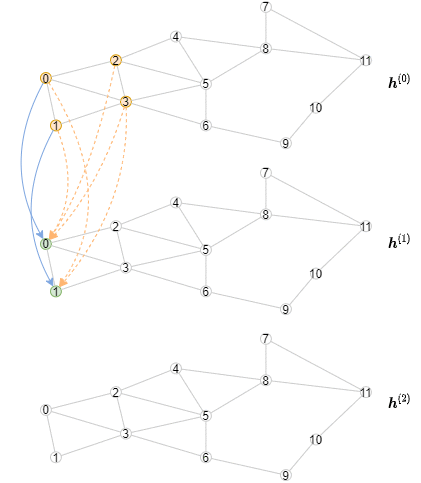# 6.6 Exact Offline Inference on Large Graphs¶

(中文版)

Both subgraph sampling and neighborhood sampling are to reduce the memory and time consumption for training GNNs with GPUs. When performing inference it is usually better to truly aggregate over all neighbors instead to get rid of the randomness introduced by sampling. However, full-graph forward propagation is usually infeasible on GPU due to limited memory, and slow on CPU due to slow computation. This section introduces the methodology of full-graph forward propagation with limited GPU memory via minibatch and neighborhood sampling.

The inference algorithm is different from the training algorithm, as the representations of all nodes should be computed layer by layer, starting from the first layer. Specifically, for a particular layer, we need to compute the output representations of all nodes from this GNN layer in minibatches. The consequence is that the inference algorithm will have an outer loop iterating over the layers, and an inner loop iterating over the minibatches of nodes. In contrast, the training algorithm has an outer loop iterating over the minibatches of nodes, and an inner loop iterating over the layers for both neighborhood sampling and message passing.

The following animation shows how the computation would look like (note that for every layer only the first three minibatches are drawn).## Implementing Offline Inference¶

Consider the two-layer GCN we have mentioned in Section 6.1 Adapt your model for minibatch training. The way to implement offline inference still involves using `MultiLayerFullNeighborSampler`, but sampling for only one layer at a time. Note that offline inference is implemented as a method of the GNN module because the computation on one layer depends on how messages are aggregated and combined as well.

```class StochasticTwoLayerGCN(nn.Module):
def __init__(self, in_features, hidden_features, out_features):
super().__init__()
self.hidden_features = hidden_features
self.out_features = out_features
self.conv1 = dgl.nn.GraphConv(in_features, hidden_features)
self.conv2 = dgl.nn.GraphConv(hidden_features, out_features)
self.n_layers = 2

def forward(self, blocks, x):
x_dst = x[:blocks.number_of_dst_nodes()]
x = F.relu(self.conv1(blocks, (x, x_dst)))
x_dst = x[:blocks.number_of_dst_nodes()]
x = F.relu(self.conv2(blocks, (x, x_dst)))
return x

def inference(self, g, x, batch_size, device):
"""
Offline inference with this module
"""
# Compute representations layer by layer
for l, layer in enumerate([self.conv1, self.conv2]):
y = torch.zeros(g.number_of_nodes(),
self.hidden_features
if l != self.n_layers - 1
else self.out_features)
g, torch.arange(g.number_of_nodes()), sampler,
batch_size=batch_size,
shuffle=True,
drop_last=False)

# Within a layer, iterate over nodes in batches
for input_nodes, output_nodes, blocks in dataloader:
block = blocks

# Copy the features of necessary input nodes to GPU
h = x[input_nodes].to(device)
# Compute output.  Note that this computation is the same
# but only for a single layer.
h_dst = h[:block.number_of_dst_nodes()]
h = F.relu(layer(block, (h, h_dst)))
# Copy to output back to CPU.
y[output_nodes] = h.cpu()

x = y

return y
```

Note that for the purpose of computing evaluation metric on the validation set for model selection we usually don’t have to compute exact offline inference. The reason is that we need to compute the representation for every single node on every single layer, which is usually very costly especially in the semi-supervised regime with a lot of unlabeled data. Neighborhood sampling will work fine for model selection and validation.

One can see GraphSAGE and RGCN for examples of offline inference.